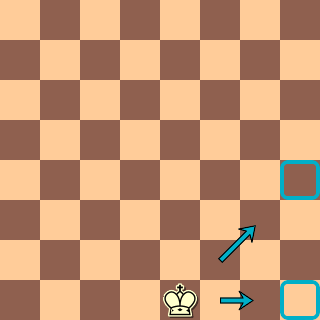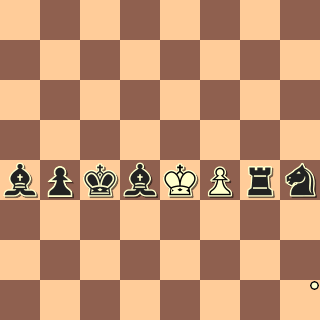# between

The `between` filter consists of the single word `between` followed by two set filters enclosed in parentheses:
```    between-filter:= 'between' '(' set-filter1 set-filter2 ')'
set-filter1 := set-filter
set-filter2 :=  set-filter```

The `between` filter represents squares that are between some line segment (horizontal, vertical or diagonal) whose endpoints are in set-filter1 and set-filter2.

More formally, the `between` filter is a set filter whose associated set in a position is the set of squares between the sets associated to set-filter1 and set-filter2. That is, square s is a member of the set associated with a `between` filter in a position if there are squares t1, t2 in the sets associated with set-filter1, setfilter2 respectively such that s is between t1 and t2. A square s is between squares t1 and t2 if

1. s is neither t1 nor t2
2. There is basic direction d such that, beginning at square t1 and traversing a path of squares in the direction d, the square s will be traversed.
For example:`between (K h1-8)` = [f1,g1,f2,g3]
Of the h-file squares, only e1-h1 and e1-h4 form a line (direction).
Only the squares `between` the endpoints of each line are part of the set.

D. Hlebec
1/2 prize, Berger 170 MT
2015```piece \$x in [Aa]
piece \$y in [Aa]
countsquares 6
[Aa] on between (\$x \$y)
```

This matches any line, orthogonal or diagonal, that has 8 pieces. One of the matches is the stalemate above.

Examples